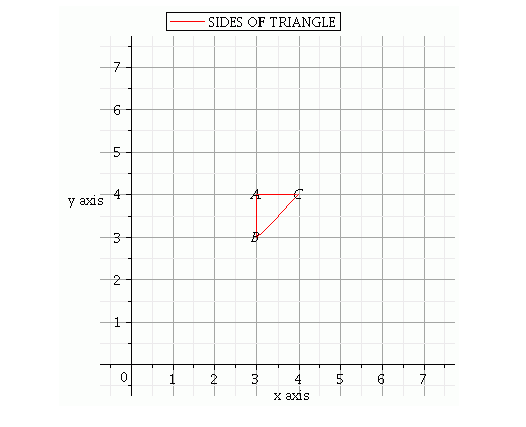# The area of the triangle formed by the lines`
Question:

The area of the triangle formed by the lines x = 3, y = 4 and x = y is

(a) $1 / 2$ sq. unit

(b) 1 sq. unit

(c) 2 sq. unit

(d) None of these

Solution:

Given $x=3, y=4$ and $x=y$

We have plotting points as $(3,4)(3,3)(4,4)$ when $x=y$Therefore, area of $\triangle A B C=\frac{1}{2}($ Base $\times$ Height $)=\frac{1}{2}(A B \times A C)=\frac{1}{2}(1 \times 1)=\frac{1}{2}$

Area of triangle $A B C$ is $\frac{1}{2}$ square units

Hence, the correct choice is a